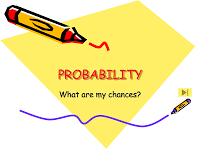# Math 7 Plus Probability Quiz

15 Questions | Total Attempts: 121SettingsStudents will test there knowledge of probability with this short online quiz.

• 1.
• A.

1/2

• B.

1/4

• C.

3/7

• D.

2/1

• 2.
You pick a card at random. Without putting the first card back, you pick a second card at random. What is P(yellow, blue)?
• A.

6/42

• B.

3/32

• C.

3/21

• D.

5/15

• 3.
You pick a marble at random. When you put the first marble back, you select a second marble at random. What is P(green, orange)?
• A.

1/11

• B.

2/3

• C.

1/12

• D.

5/48

• 4.
You spend a spinner thrice. What is the probability of P(5, even number, and 1)
• A.

1/9

• B.

8/864

• C.

5/36

• D.

1/108

• 5.
Landing on heads after tossing a coin and rolling a 5 on a single 6-sided die
• A.

Independent Events

• B.

Dependent Events

• 6.
A bag contains 3 red cubes, 4 green cubes, and 5 blue cubes. One cube is taken from the bag and is not replaced. Another cube is taken.
• A.

Dependent

• B.

Independent

• 7.
Spinning a number 6 and then spinning a number 5 on the same spinner.
• A.

Independent Events

• B.

Dependent Events

• 8.
A spinner numbered 1 through 10 is spun 100 times. The results of the experiment are shown in the table below. What is the experimental probability of spinning an 8?
• A.

7/100

• B.

1/100

• C.

13/100

• D.

10/100

• 9.
From left to right on the number line, order the events based on how likely they are to occur.a. The event is certain to happen.b. The event is just as likely to happen as not to happen.c. The event has no chance of happening.d. The event could happen but is unlikely.e. The event is likely to happen.
• A.

A, e, b, d, c

• B.

D, c, b, e, a

• C.

D, c, b, a, e

• D.

C, d, b, e, a

• 10.
A set of 15 cards is numbered 1, 2, 3, …, 15. Suppose you choose one card at random without looking. What is the probability of choosing an odd-numbered card?
• A.

1/2

• B.

7/15

• C.

1/15

• D.

8/15

• 11.
Something that is unlikely to happen has a probability of between
• A.

1 and 2

• B.

0.5 and 1

• C.

0 and 0.5

• 12.
A bag contains just 5 buttons, all of which are blue. What is the probability of picking a red button from the bag?
• A.

0.5

• B.

1

• C.

0

• 13.
A bag contains 4 white buttons. How many black buttons must be added so there is an even chance of picking a white button?
• A.

8

• B.

4

• C.

2

• D.

0

• 14.
You have 15 pennies in your pocket. Of those pennies, 3 are Canadian. Suppose you pick a penny out of your pocket at random. Find P(not Canadian).  This is a ___________________________event.
• 15.
M A T H E M A T I C S ( Each letter represents a card)You select a card at random. Without replacing the card, you select a second card. Find the probability.  P(M, then H)
Related TopicsBack to top